GeeksforGeeks App
Open AppBrowser
Continue

# How to Use Solver in Excel?

A solver is a mathematical tool present in MS-Excel that is used to perform calculations by working under some constraints/conditions and then calculates the solution for the problem. It works on the objective cell by changing the variable cells any by using sum constraints.

Solver is present in MS- Excel but for using it we need to activate it. For activating the solver tool we need to do the following steps:

Step 1: Go to File and select options. The following dialog box will appear.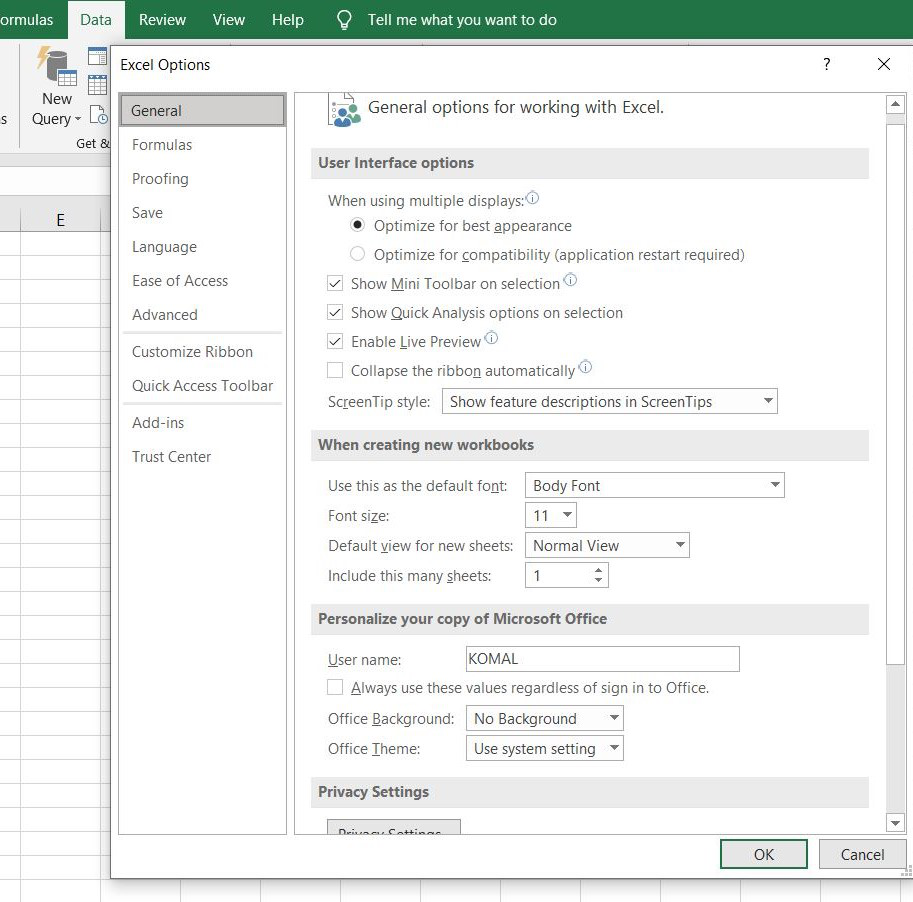Step 2: Now select the Add-ins option and click on Go and finally click on OK.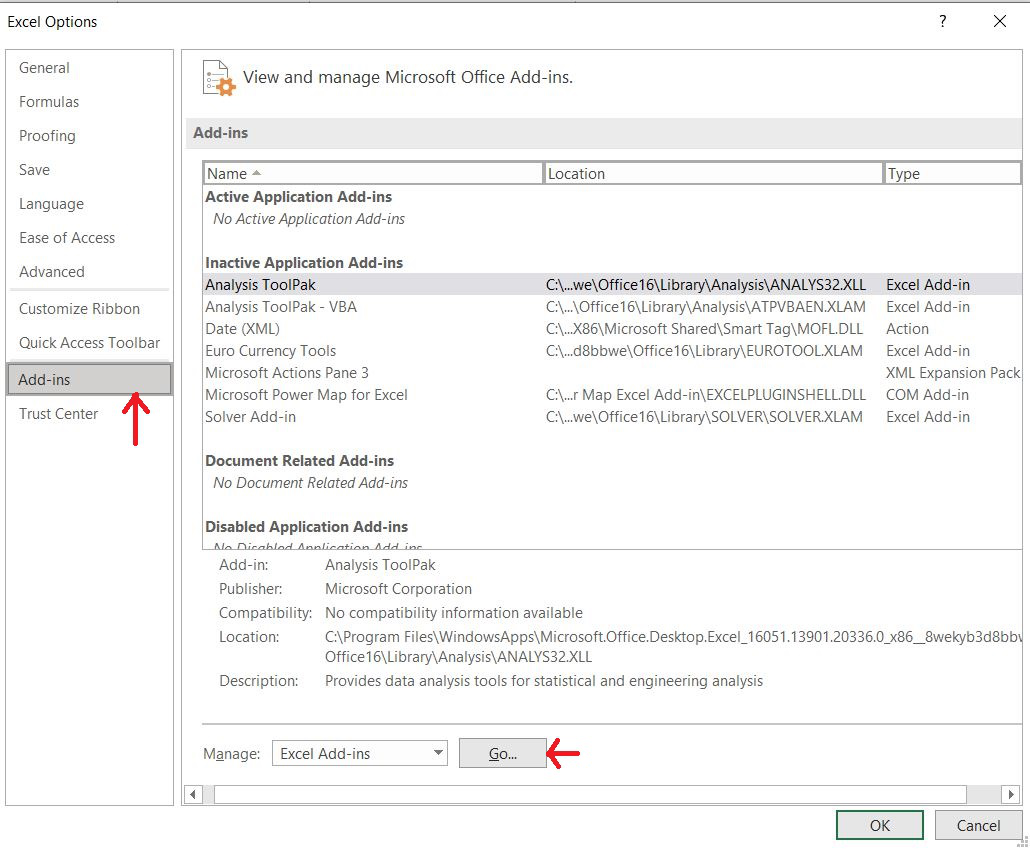Step 3: After clicking OK, Select Solver Add-in and press OK. Now solver will be activated in Excel.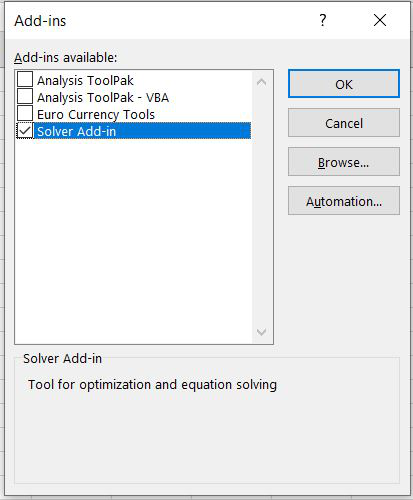Step 4: Now solver will appear in data section like this.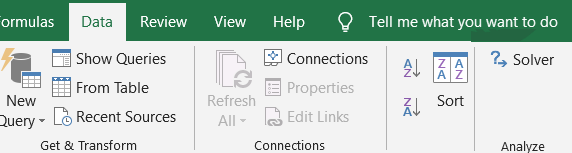Now let’s understand how to use solver with the help of an example.

Example:

We went to a mall and we also have a gift voucher worth rs.10,000 and We want to purchase items in such a manner that all the money of the gift voucher gets utilized.

So, suppose we purchased the following items.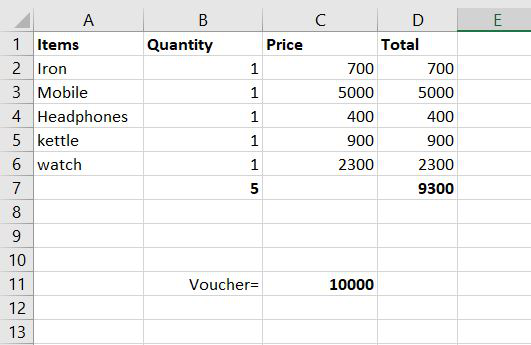Suppose we purchased the above items in only one quantity and the total came out to be 9300 but the voucher was for rs.10,000. So now we want to use Solver for this purpose. Now let’s see how it will be done.

Step 1: Firstly go on data and find solver there and click it. The following dialog box will appear. Now in this, we have to select the objective in which we want to change our value.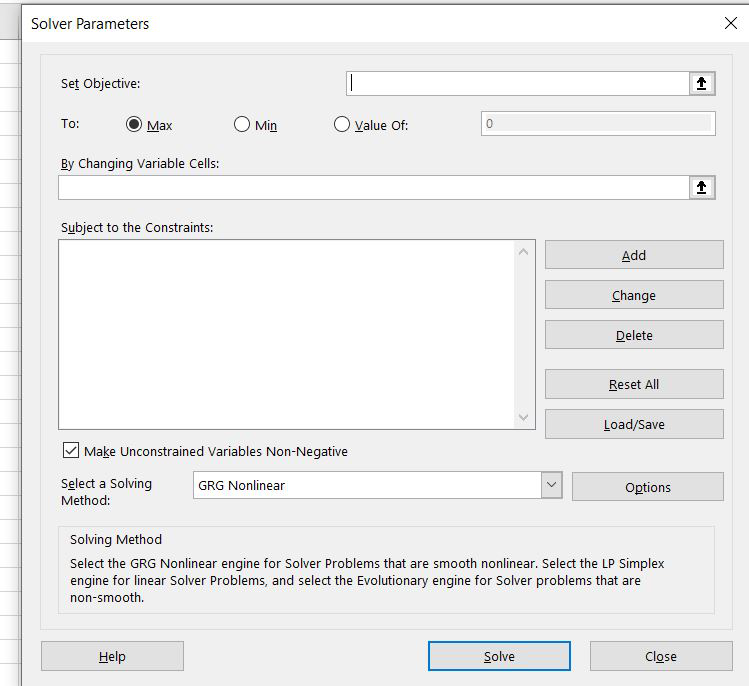Step 2: In the set objective we have to select the total of the D column because want the value to change from 9300 to 10,000. After clicking on D7 following thing will be displayed on the set objective block.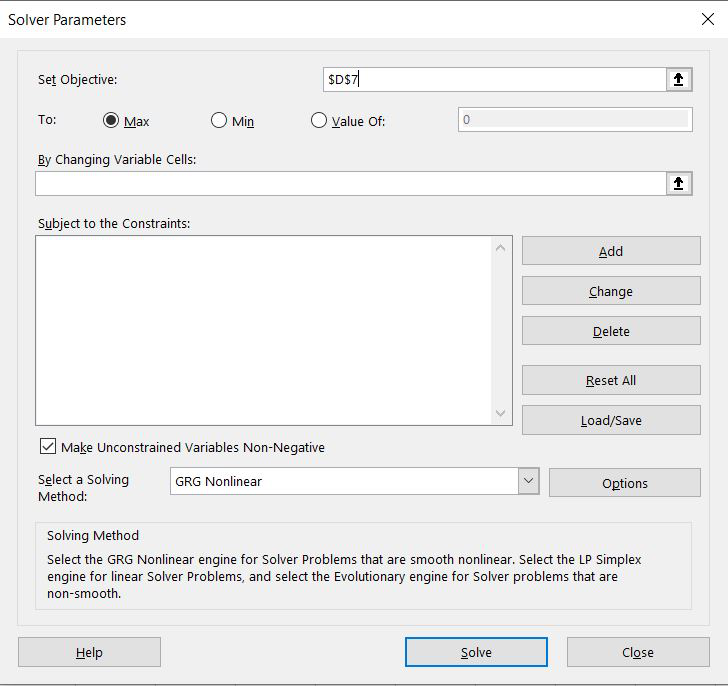Step 3: Now in the ‘By changing Variable cell’ we will select the Quantity cell because we want to change the quantity in such a way so that the total amount comes to 10,000.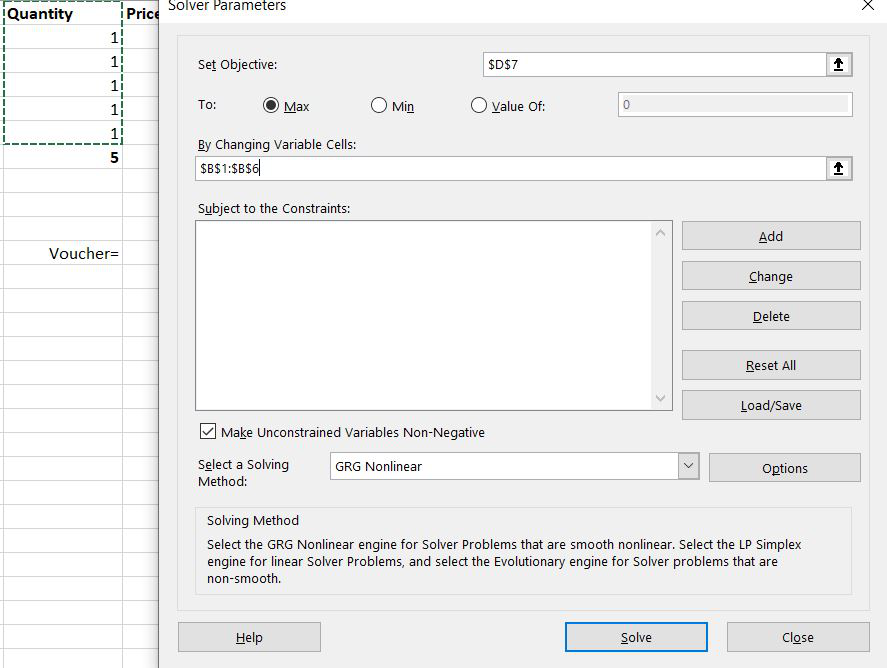Step 4: Now we have to set some conditions under which we want our work to get done. So for setting some conditions /constraints, we will click on Add.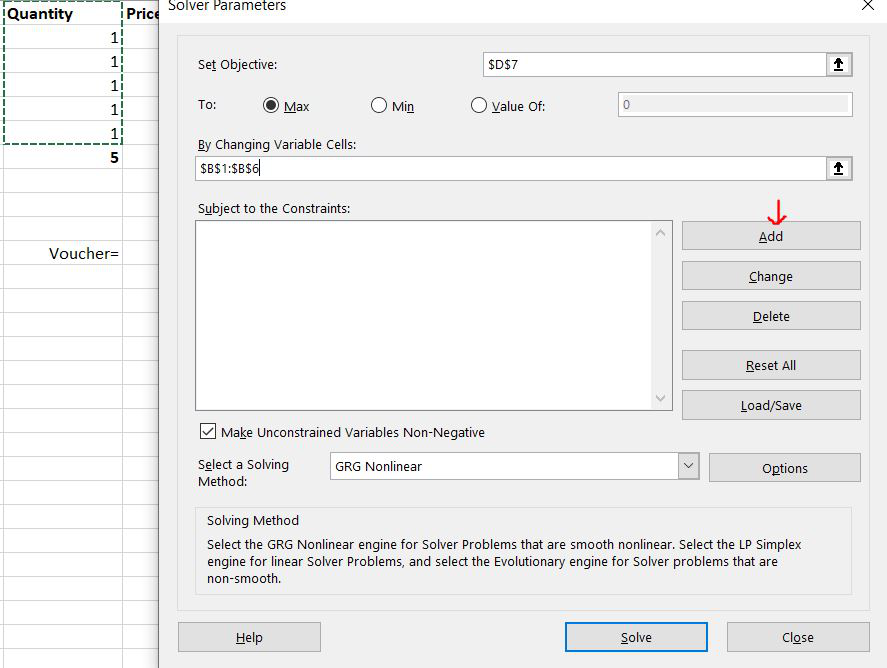Step 5– Now, a dialog box will appear and we will add 3 conditions. The first condition is that the total amount should be equal to the voucher amount. So we will select cell D7 in a cell reference and then = sign, and finally, we will select cell C11. Now the first condition is added. To add the next condition press Add.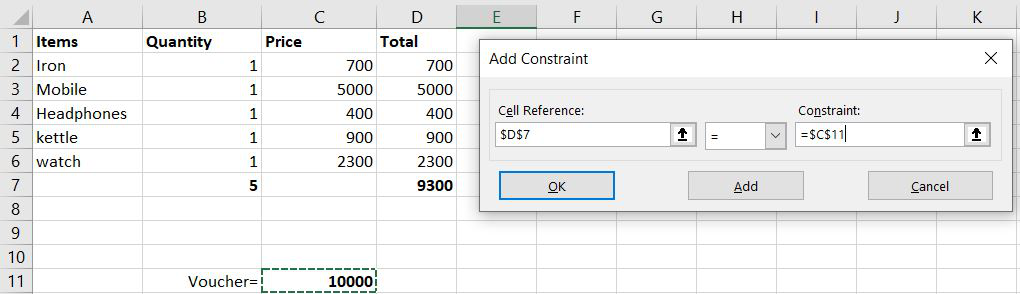Step 6: For the second condition we will select the Quantity cell because we want the quantity to be an integer value, a whole value. So in cell reference, we will select from B2 to B6 then int, and then again will press Add.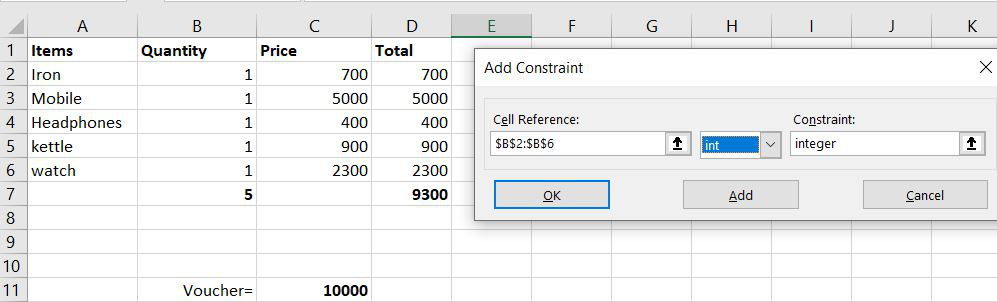Step 7- Now for the third condition, we want that our item quantity should never be un negative which is not possible in real life. So we will select cells from B2 to B6 and should be >= to zero. Then click Add and cancel.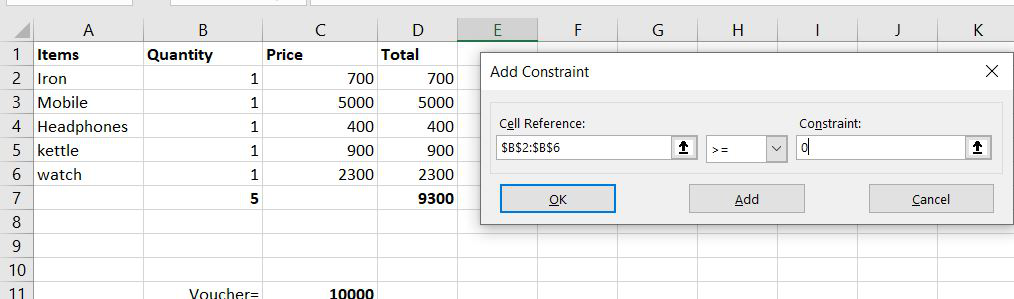Step 8: Now the following dialog box will show all the 3 conditions that we used and now click on Solve.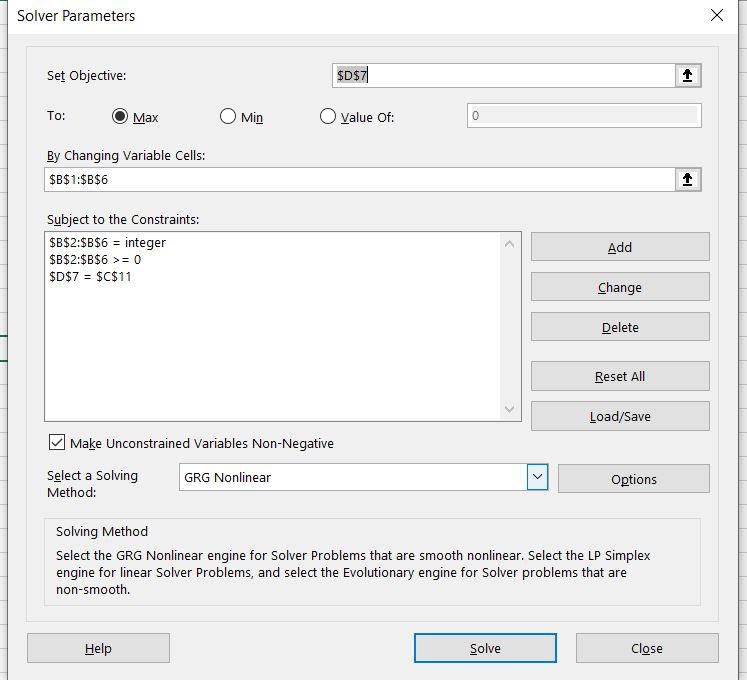Step 9: By clicking Solve the solver will provide the desired output and to keep the answer we will click on keep solver solution.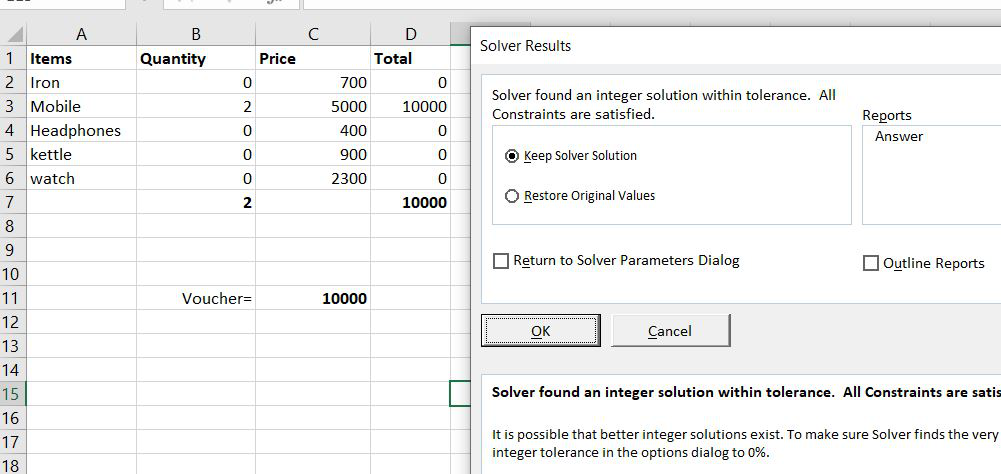So, this is what a solver is, How it is activated by not by default present in Excel and this is how it is used.

My Personal Notes arrow_drop_up